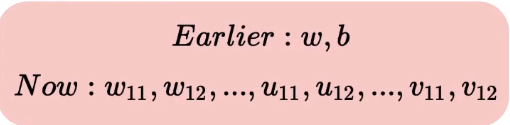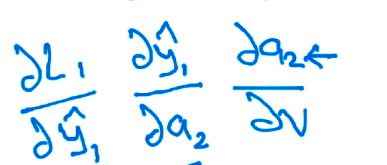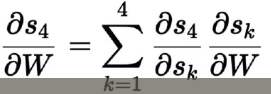# Learning Algorithm - Recurrent Neural Networks(RNNs)

## Learning Algorithm

One question at this point would be “can the gradient descent algorithm be used in the context of a recurrent neural network?There would be bias terms as well in addition to “w”, “v”

## Learning Algorithm — Derivatives w.r.t. V

Let’s briefly look at how the derivatives would be computed for backpropagation steps as part of the learning algorithma₂ is the above image refers to the pre-activation at layer 2

## Learning Algorithm — Derivatives w.r.t. W

This is what the network looks like where the “model predicts an output at each time step” and “loss is also computed at each time step” using the cross-entropy loss functionHere 4 represent the number of time steps

--

--